## Runge Kutta-4

So far, Fourth Order Runge-Kutta is the most popular and widely used. For many practical purposes, you may use this solution.

Suppose we have ODE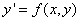then

Formula :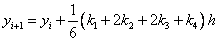Where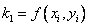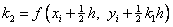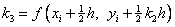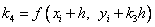Error term :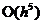, correct up to the third order term in Taylor series expansion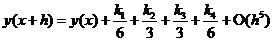Example:, set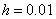, with initial condition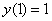. The few first results and the graph of solution are given below.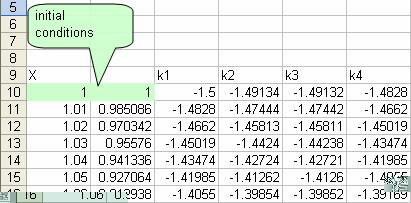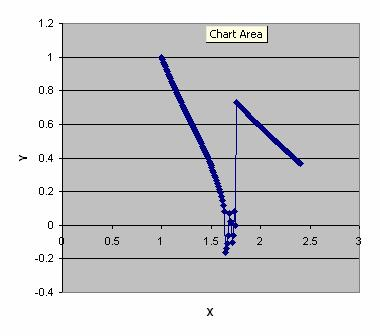Note that after, the solution is not correct. See Comparison .

Preferable reference for this tutorial is

Teknomo, Kardi (2015) Solving Ordinary Differential Equation (ODE). https:\\people.revoledu.com\kardi\tutorial\ODE\